• 将所有询问建成一颗平衡树 ...暴力合并的复杂度怎么证呢 可以通过与prize的关系 发现右边的数减去prize后肯定小于原来的一半  每个数最多从右边到左边log次 复杂度就是nlogn了 #include #include #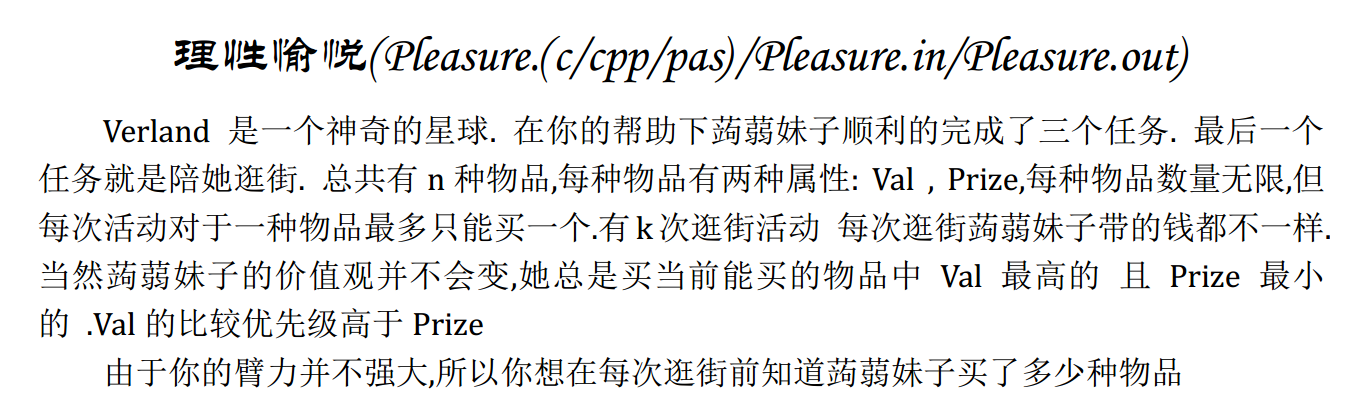将所有询问建成一颗平衡树

每次分裂成两棵  右边打个减标记

然后怎么合并呢

要是右边最小值大于左边最大值 那么直接合并

要是不呢 我们不断从右边弹出最小插入左边 直到可以合并

暴力合并的复杂度怎么证呢

可以通过与prize的关系 发现右边的数减去prize后肯定小于原来的一半

每个数最多从右边到左边log次

复杂度就是nlogn了

#include<cstdio>
#include<cstdlib>
#include<algorithm>
using namespace std;
typedef long long ll;

inline char nc(){
static char buf,*p1=buf,*p2=buf;
if (p1==p2) { p2=(p1=buf)+fread(buf,1,100000,stdin); if (p1==p2) return EOF; }
return *p1++;
}

char c=nc(),b=1;
for (;!(c>='0' && c<='9');c=nc()) if (c=='-') b=-1;
for (x=0;c>='0' && c<='9';x=x*10+c-'0',c=nc()); x*=b;
}

char c=nc(),b=1;
for (;!(c>='0' && c<='9');c=nc()) if (c=='-') b=-1;
for (x=0;c>='0' && c<='9';x=x*10+c-'0',c=nc()); x*=b;
}

const int N=400005;

struct node{
node *l,*r;
int idx,ans,fla;
int fix,size; ll tag,key;
node (){}
node (ll key,int idx,node *null):fla(0),ans(0),idx(idx),l(null),r(null),tag(0),fix(rand()*rand()),key(key),size(1){}
void Tag(ll t,int f){
tag+=t; key-=t;
fla+=f; ans+=f;
}
void pushdown(){
if (fla){
l->Tag(tag,fla);
r->Tag(tag,fla);
tag=0; fla=0;
}
}
void update(){
size=l->size+r->size+1;
}
}nodes[N],*root,*null;

typedef pair<node*,node*> Droot;

node *M(node *A,node *B){
if (A==null) return B;
if (B==null) return A;
A->pushdown(); B->pushdown();
if (A->fix<B->fix){
A->r=M(A->r,B); A->update();
return A;
}else{
B->l=M(A,B->l); B->update();
return B;
}
}

Droot Split(node *x,int k){
if (x==null) return Droot(null,null);
Droot y; x->pushdown();
if (x->l->size>=k){
y=Split(x->l,k);
x->l=y.second; x->update();
y.second=x;
}else{
y=Split(x->r,k-x->l->size-1);
x->r=y.first; x->update();
y.first=x;
}
return y;
}

node *Build(pair<ll,int> *a,int n){
static node *stack[N],*x,*last;
int pnt=0;
null=nodes; null->l=null->r=null; null->size=0;
for (int i=1;i<=n;i++){
nodes[i]=node(a[i].first,a[i].second,null);
x=nodes+i;
last=null;
while (pnt && stack[pnt]->fix>x->fix)
stack[pnt]->update(),last=stack[pnt--];
if (pnt) stack[pnt]->r=x;
x->l=last;
stack[++pnt]=x;
}
while (pnt) stack[pnt--]->update();
return stack;
}

inline int Getkth(node *x,ll v){
if (x==null) return 0;
x->pushdown();
return x->key<v?Getkth(x->r,v)+x->l->size+1:Getkth(x->l,v);
}

inline node *Insert(node *rt,node *v){
int k=Getkth(rt,v->key);
Droot x=Split(rt,k);
return M(M(x.first,v),x.second);
}

inline ll Max(node *x){
x->pushdown();
return x->r==null?x->key:Max(x->r);
}
inline ll Min(node *x){
x->pushdown();
return x->l==null?x->key:Min(x->l);
}

struct abcd{
int v,p;
bool operator < (const abcd &B) const{
return v==B.v?p<B.p:v>B.v;
}
}W[N];

int n,K;
pair<ll,int> a[N];

inline void Solve(int prize){
int k=Getkth(root,prize);
if (!k){
root->Tag(prize,1);
return;
}
Droot x=Split(root,k);
x.second->Tag(prize,1);
ll maxv=Max(x.first),minv=Min(x.second);
while (maxv>minv){
Droot y=Split(x.second,1);
x.first=Insert(x.first,y.first);
x.second=y.second;
minv=Min(x.second);
}
root=M(x.first,x.second);
}

int Ans[N];

inline void Print(node *x){
if (x==null) return;
x->pushdown();
Ans[x->idx]=x->ans;
Print(x->l);
Print(x->r);
}

int main(){
freopen("pleasure.in","r",stdin);
freopen("pleasure.out","w",stdout);
for (int i=1;i<=n;i++)
sort(W+1,W+n+1);
++K; a[K]=make_pair(1LL<<60,K);
sort(a+1,a+K+1);
root=Build(a,K);
for (int i=1;i<=n;i++)
Solve(W[i].p);
Print(root);
for (int i=1;i<K;i++)
printf("%d ",Ans[i]);
return 0;
}

展开全文• treap模版

千次阅读 2017-01-16 20:28:37
Treap 的随机优先级不同,它们维护的附加域要动态的调整,而 Treap 的随机修正值一经生成不再改变,这一点使得灵活性不如 Treap。 AVL 和红黑树都是时间效率很高的经典算法,在许多专业的应用领域(如 STL)有着十分...

之前我们讲到二叉搜索树，从二叉搜索树到2-3树到红黑树到B-树。
二叉搜索树的主要问题就是其结构与数据相关，树的深度可能会很大，Treap树就是一种解决二叉搜索树可能深度过大的另一种数据结构

Treap

Treap=Tree+Heap。Treap本身是一棵二叉搜索树，它的左子树和右子树也分别是一个Treap，和一般的二叉搜索树不同的是， Treap纪录一个额外的数据，就是优先级。Treap在以关键码构成二叉搜索树的同时，还满足堆的性质。 这些优先级是是在结点插入时，随机赋予的，Treap根据这些优先级满足堆的性质。这样的话，Treap是有一个随机附加域满足堆的性质的二叉搜索树，其结构 相当于以随机数据插入的二叉搜索树。其基本操作的期望时间复杂度为O(logn)。相对于其他的平衡二叉搜索树，Treap的特点是实现简单，且能基本实现随机平衡的结构。
Treap维护堆性质的方法 只用到了旋转，只需要两种旋转，编程复杂度比Splay要小一些。

插入

给节点随机分配一个优先级，先和二叉搜索树的插入一样，先把要插入的点插入到一个叶子上，然后 跟维护堆一样，如果当前节点的优先级比根大就旋转，如果 当前节点是根的左儿子就右旋，如果当前节点是根的右儿子就左旋

下面图解旋转操作：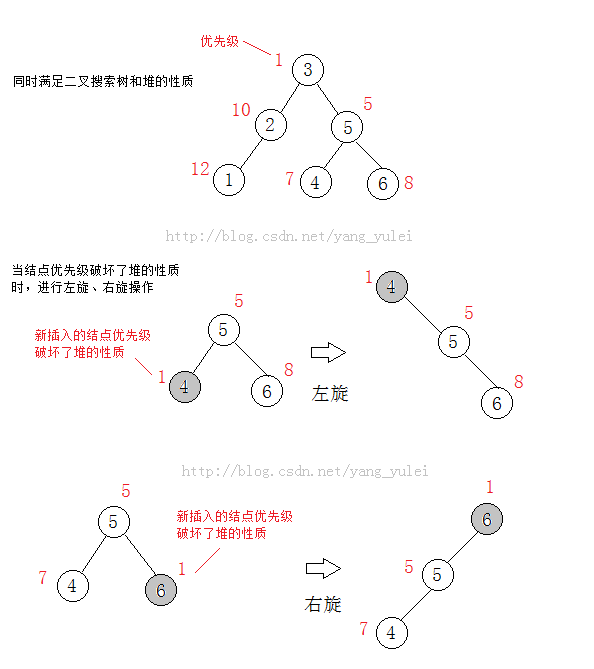由于旋转是O(1)的，最多进行h次（h是树的高度），插入的复杂度是O(h)的，在期望情况下h=O(logn)，所以它的期望复杂度是O(logn)。

下面我们以一个 实例来图解Treap的插入过程，看完之后你一定对Treap的插入了然于胸。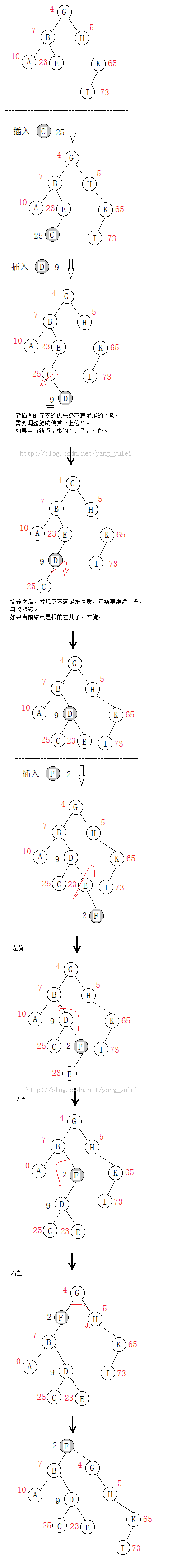删除

删除一个节点有两种方式，可以像删除二叉树节点那样删除一个节点，也可以像删除堆中节点那样删除。

1、用二叉搜索树的方式删除
先在二叉查找树中找到要删除的节点的位置,然后根据节点分以下情况:
情况一:
该节点是 叶节点(没有非空子节 点的节点),直接把节点删除即可。

情况二：
该节点是 链节点(只有一个非空子节点的节点),为了删除这个节点而不影响它的子树,需要把它的子节点代替它的位置,然后把它删除。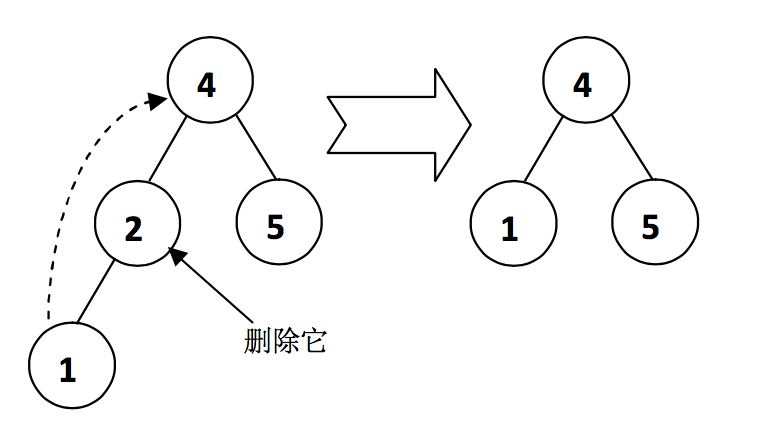情况三：
该节点 有两个非空子节点。由于情况比较复杂,一般的策略是 用它右子树的最小值来代替它,然后把它删除。如图所示,删除节点2时,在它的右子树中找到最小的节点3, 该节点一定为待删除节点的后继。删除节点3(它可能是叶节点或者链节点),然后把节点2的值改为3。
（当然，我们也可以使用结点的左子树的最大值来替代它， 为了不使Treap向一边偏沉,我们可以随机地选取是用后继还是前驱代替它, 并保证两种选择的概率均等。）关于查找最小值：
基本方法就是从子树的根节点开始, 如果左子节点不为空,那么就访问左子节点,直到左子节点为空,当前节点就是该子树的最 小值节点。删除它只需用它的右子节点代替它本身。

2、用堆的方式删除

因为Treap满足堆性质，所以只需要把要删除的节点旋转到叶节点上，然后直接删除就可以了。
具体的方法：
如果该节点的左子节点的优先级小于右子节点的优先级，右旋该节点,使该节点降为右子树的根节点，然后访问右子树的根节点,继续操作；

反之，左旋该节点,使该节点降为左子树的根节点，然后访问左子树的根节点，继续操作，直到变成可以直接删除的节点。
（即：让小优先级的结点旋到上面，满足堆的性质）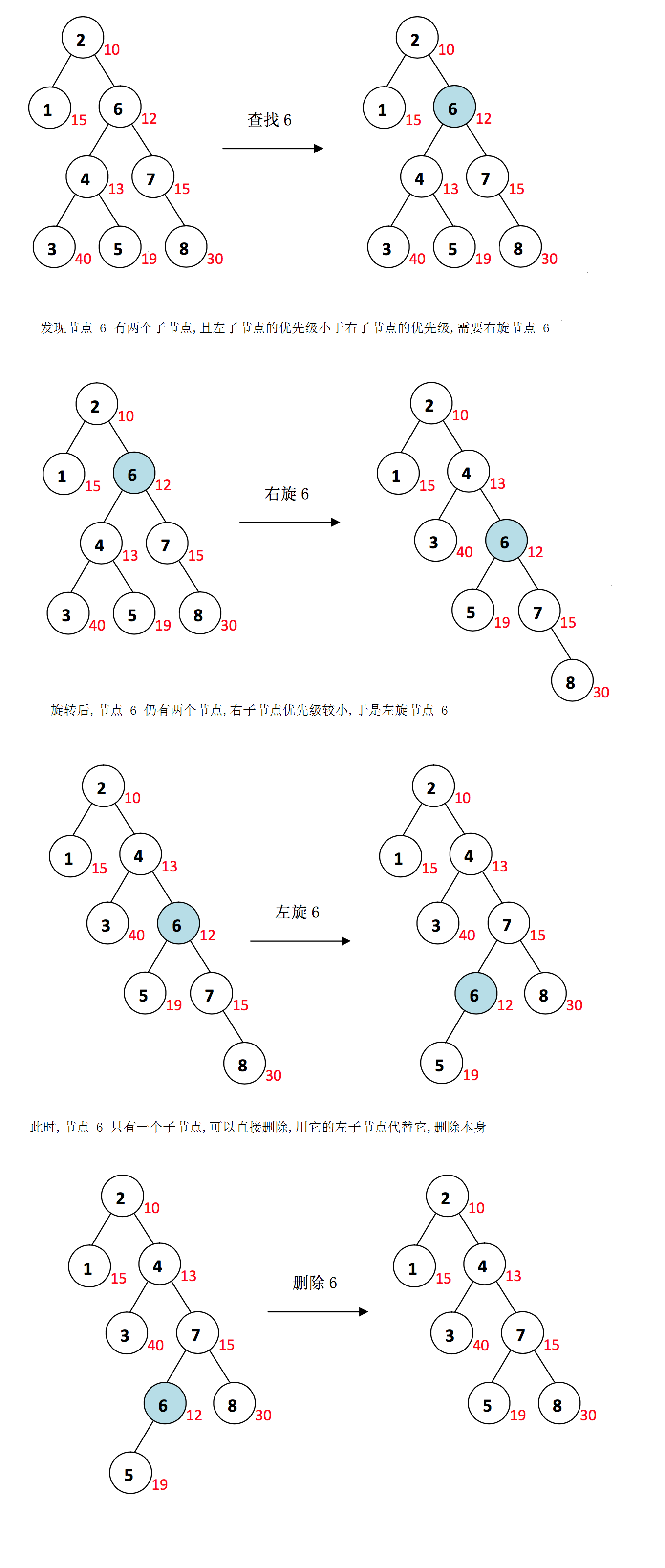删除最多进行O(h)次旋转，期望复杂度是O(logn)。

查找

和一般的二叉搜索树一样，但是由于Treap的随机化结构，Treap中查找的期望复杂度是O(logn)。

对比

与 Splay树 相比:
Splay 和 BST 一样,不需要维护任何附加域,比 Treap 在空间上有节约。但 Splay 在查找时也会调整结构,这使得 Splay 灵活性稍有欠缺。 Splay 的查找插入删除等基本操作的时间复杂度为均摊O(logN)而非期望。可以故意构造出使 Splay 变得很慢的数据。

与AVL 红黑树相比：
AVL 和红黑树在调整的过程中,旋转都是均摊 O(1)的,而 Treap 要 O(logN)。与 Treap 的随机优先级不同,它们维护的附加域要动态的调整,而 Treap 的随机修正值一经生成不再改变,这一点使得灵活性不如 Treap。
AVL 和红黑树都是时间效率很高的经典算法,在许多专业的应用领域(如 STL)有着十分重要的地位。然而AVL和红黑树的编程实现的难度要比Treap大得多。

维护子树的大小

Treap 是一种排序的数据结构,如果我们想 查找第k小的元素 或者 询问某个元素在Treap中从小到大的排名 时,我们就必须知道每个子树中节点的个数。
由于插入、删除、旋转等操作,会使每个子树的大小改变,所 以我们必须对子树的大小进行动态的维护。

对于 旋转 ,我们要在旋转后对子节点和根节点分别重新计算其子树的大小。
对于 插入 ,在寻找插入的位置时,每经过一个节点,都要先使以它为根的子树的大小增加 1,再递归进入子树查找。
对于 删除 ,在寻找待删除节点,递归返回时要把所有的经过的节点的子树的大小减少 1。要注意的是,删除之前一定要保证待删除节点存在于 Treap 中。

（维护了子树的大小，我们就可以求“ 排名第k的元素 ”这样的问题了。快排也能求“第k大”问题，但是快排适合静态的数据，对于经常变动的数据，我们用树结构来维护更灵活。）
（我们还可以求“ 某个元素的排名 ”，我们的基本思想是查找目标元素在 Treap 中的位置,且在查找路径中统计出小于目标元素的节点的总个数,目标元素的排名就是总个数+1。即：在查找的路径中统计小于目标元素的个数，当找到目标元素后加上其左子树的个数即可。）

举个栗子

Treap 是一种高效的动态的数据容器,据此我们可以用它处理一些数据的动态统计问题。

一、一个应用实例
[问题描述] 有一个游戏排名系统,通常要应付三种请求:上传一条新的得分记录、查询某个玩家的当前 排名以及返回某个区段内的排名记录。当某个玩家上传自己最新的得分记录时,他原有的得 分记录会被删除。为了减轻服务器负担,在返回某个区段内的排名记录时,最多返回 10 条 记录。
[求]
（1）更新玩家的得分记录
（2）查询玩家排名（如果两个玩家的得分相同, 则先得到该得分的玩家排在前面。）
（3）查询第 Index 名开始的最多 10 名玩家名字

[解]
因为作为字符串的姓名是不便于处理的,我们给每个玩家都制定一个ID,首先要建立一个由姓名到玩家ID的映射数据结构。为了查找快速,可以用Trie树。之后我们建立一个双关键字的Treap,关键字1为得分从小到大,关键字2为时间戳从大到小,这种排列方式的逆序,恰好是我们要的顺序(也可以直接就是逆序)。

对于问题（1），先查询玩家是否已经存在,如果已经存在,在Treap中更新对应已经存在的记录。
对于问题（2），就是基本的求排名操作。
对于问题（3），就是分别查找第(总记录数 + 1 – k)小的记录。

二、双端优先队列的实现
优先队列(Priority Queue)是一种按优先级维护进出顺序的数据容器结构,可以选择维护实现取出最小值或最大值。我们通常用堆实现优先队列,通常取出最值的时间复杂度为 O(logN)。
用最小堆可以实现最小优先队列,用最大堆可以实现最大优先队列。但是如果我们要求一种 “双端优先队列”,即要求同时支持插入、取出最大值、取出最小值的操作,用一个单纯的堆就不能高效地实现了。
（可以用两个堆来实现，两堆中的元素都互指，但维护两个堆比较复杂。）

我们可以方便地使用Treap实现双端优先队列,只需建立一个 Treap,分别写出取最大值和最小值的功能代码就可以了, 无需做任何修改。由于Treap平衡性不如堆完美,但期望时间仍是 O(logN)。更重要的是在 实现的复杂程度上大大下降,而且便于其他操作的推广。所以,用 Treap 实现优先队列不失为一种便捷而又灵活的方法。

其它：

平衡树并不适合作为所有数据类型的数据的有序存储容器,因为可能有些类型的两个元素直接相互比较大小是十分 耗时的,这个常数时间的消耗是无法忍受的。例如字符串,作为检索字符串的容器,我们更推荐Trie树,而不是平衡树。平衡树仅适合做元素间相互比较时间很少的类型的有序存储容器。

关于查找最小值：
基本方法就是从子树的根节点开始, 如果左子节点不为空,那么就访问左子节点,直到左子节点为空,当前节点就是该子树的最 小值节点。删除它只需用它的右子节点代替它本身。

【参考】

中文维基百科http://zh.wikipedia.org/wiki/%E6%A0%91%E5%A0%86
《随机平衡二叉查找树Treap的分析与应用》 清华大学计算机系 郭家宝

#include<iostream>
#include<cstring>
#include<algorithm>
#include<cstdio>
#include<cmath>
#include<queue>
#include<vector>
#include<climits>
#include<string>
#include<cstdlib>
#include<set>
#include<stack>
#include<map>
#include<bitset>
#include<ctime>
using namespace std;
typedef int ll;
typedef unsigned long long ull;
{
char k=0;char ls;ls=getchar();for(;ls<'0'||ls>'9';k=ls,ls=getchar());
ll x=0;for(;ls>='0'&&ls<='9';ls=getchar())x=x*10+ls-'0';
if(k=='-')x=0-x;return x;
}
struct E{
ll lc,rc;//左右孩子
ll s,sum;//权值和随机数
ll fa;//父亲
ll size;//子节点大小
ll js;//该节点元素计数
}t;
ll w;
ll size;
ll root;

inline ll find(ll s)//查找
{
if(size==0)
return 0;
ll now=root;
while(now)
{
if(t[now].s==s)
return now;
if(s<t[now].s)
{
now=t[now].lc;
continue;
}
if(s>t[now].s)
{
now=t[now].rc;
continue;
}
}
return 0;
}

ll maxn()//最大值
{
ll now=root;
while(1)
{
if(t[now].rc==0)
return now;
now=t[now].rc;
}
}

ll minn()//最小值
{
ll now=root;
while(1)
{
if(t[now].lc==0)
return now;
now=t[now].lc;
}
}

void update(ll x)//更新平衡树尺寸
{
ll now=root;
while(1)
{
--t[now].size;
if(now==x)return;
if(t[x].s<t[now].s)
{
now=t[now].lc;
continue;
}
if(t[x].s>t[now].s)
{
now=t[now].rc;
continue;
}
}
return;
}

void zig(ll x)//左旋
{
t[t[x].fa].size-=t[t[x].rc].size;
t[t[x].fa].size-=t[x].js;
t[x].size+=t[t[t[x].fa].lc].size;
t[x].size+=t[t[x].fa].js;
if(root==t[x].fa)
root=x;
ll ff=t[t[x].fa].fa;
t[t[x].fa].fa=x;
if(ff!=0)
{
if(t[ff].lc==t[x].fa)
t[ff].lc=x;
if(t[ff].rc==t[x].fa)
t[ff].rc=x;
}
if(t[x].lc!=0)
t[t[x].lc].fa=t[x].fa;
t[t[x].fa].rc=t[x].lc;
t[x].lc=t[x].fa;
t[x].fa=ff;
return;
}

void zag(ll x)//右旋
{
t[t[x].fa].size-=t[t[x].lc].size;
t[t[x].fa].size-=t[x].js;
t[x].size+=t[t[t[x].fa].rc].size;
t[x].size+=t[t[x].fa].js;
if(root==t[x].fa)
root=x;
ll ff=t[t[x].fa].fa;
t[t[x].fa].fa=x;
if(ff!=0)
{
if(t[ff].lc==t[x].fa)
t[ff].lc=x;
if(t[ff].rc==t[x].fa)
t[ff].rc=x;
}
if(t[x].rc!=0)
t[t[x].rc].fa=t[x].fa;
t[t[x].fa].lc=t[x].rc;
t[x].rc=t[x].fa;
t[x].fa=ff;
return;
}

void strike_off(ll x)//删除指定下标
{
while(t[x].lc!=0||t[x].rc!=0)
{
if(t[x].lc!=0&&t[x].rc==0)
{zag(t[x].lc);continue;}

if(t[x].rc!=0&&t[x].lc==0)
{zig(t[x].rc);continue;}

if(t[x].lc!=0&&t[x].rc!=0)
{
if(t[t[x].lc].sum<t[t[x].rc].sum)
zag(t[x].lc);
else
zig(t[x].rc);
}
}
update(x);
if(t[x].fa!=0)
{
if(t[t[x].fa].lc==x)
t[t[x].fa].lc=0;
if(t[t[x].fa].rc==x)
t[t[x].fa].rc=0;
}
--size;
if(size==0)
root=0;
return;
}

void delete_(ll s)//删除指定数字
{
ll x=find(s);
if(x==0)
return;
if(t[x].js>1)
{
--t[x].js;
--size;
update(x);
return;
}
else
strike_off(x);
return;
}

void insert(ll s)//插入
{
++size;
t[++w].s=s;
t[w].sum=rand()%1000050;
t[w].size=1;
t[w].js=1;
if(size==1)
{
root=w;
return;
}
ll pre=root;
ll now=root;
while(now!=0)
{
if(s==t[now].s)
{
++t[now].size;
++t[now].js;
--w;
return;
}
if(s<t[now].s)
{
++t[now].size;
pre=now;
now=t[now].lc;
continue;
}
if(s>t[now].s)
{
++t[now].size;
pre=now;
now=t[now].rc;
continue;
}
}
if(s<t[pre].s)
t[pre].lc=w;
if(s>t[pre].s)
t[pre].rc=w;
t[w].fa=pre;

while(t[w].sum<t[t[w].fa].sum)
{
if(t[t[w].fa].lc==w)
{
zag(w);
continue;
}
if(t[t[w].fa].rc==w)
{
zig(w);
continue;
}
}
return;
}

int main()
{
srand(19981017);
return 0;
}

展开全文数据结构 平衡树 acm treap
• 介绍树堆，在数据结构中也称Treap，是指有一个随机附加域满足堆的性质的二叉搜索树，其结构相当于以随机数据插入的二叉搜索树。其基本操作的期望时间复杂度为O(logn)。对于插入给节点随机分配一个优先级，先和二叉...

介绍

树堆，在数据结构中也称Treap，是指有一个随机附加域满足堆的性质的二叉搜索树，其结构相当于以随机数据插入的二叉搜索树。其基本操作的期望时间复杂度为 O ( log ⁡ 2 n ) O(\log_2n)

对于插入

给节点随机分配一个优先级，先和二叉排序树的插入一样，先把要插入的点插入到一个叶子上，然后跟维护二叉平衡树一样，如果当前节点的优先级比父亲大就旋转，如果当前节点是父亲的左儿子就右旋，如果当前节点是父亲的右儿子就左旋。
通常来说，优先级是伴随着访问次数来改变的。

证明

在这里我们先假设优先级的分布是稠密的。
如果不是稠密的，可以通过映射来构造一个稠密的分布：
把优先级从小到大排序，然后把优先级直接用序号代替。这样就得到了一个稠密的分布
设我们要插入的节点的优先级为 X X ，treap里面最大的优先级为 L i m i t Limit ，简称 L L
首先，如果 X ≥ L X \ge L ,那么就不用旋转。
所以我们讨论 X ≤ L X \le L 的情况

我们可以得到树高
H ≈ log ⁡ 2 L H \approx \log_{2}{L}
而我们的 X X 的目标树高为
H x ≈ log ⁡ 2 X H_x \approx \log_{2}{X}
每次旋转,X都会减少一层树高（升上去了）
所以我们的旋转次数
S p i n = H − H x ≈ log ⁡ 2 L − log ⁡ 2 X Spin=H -H_x \approx \log_{2}{L} - \log_{2}{X}
由于我们的X是在 [ 0 , L ] [ 0,L] 内等可能的
那么期望旋转次数为加权平均数
∑ X = 0 L 1 L ∗ ( log ⁡ 2 L − log ⁡ 2 X ) \sum_{X=0}^L \frac{1}{L}*(\log_{2}{L} - \log_{2}{X})

≈ 1 L ∫ 0 L ( log ⁡ 2 L − log ⁡ 2 X ) d X \approx\frac{1}{L} \int_0^L(\log_{2}{L} - \log_{2}{X}) d{X}

= 1 L ln ⁡ 2 [ X ln ⁡ L − X ln ⁡ X + X ] 0 L =\frac{1}{L \ln{2}} [X\ln{L} -X\ln{X} +X]_0^L
= 1 ln ⁡ 2 − 0 = 1.44 =\frac{1}{\ln{2}}-0=1.44
证明完毕.

展开全文数据结构 搜索 结构 数据
• fhq treap

2019-06-16 17:04:18
旋转！合并！easy！quickly！ 上代码 // luogu-judger-enable-o2 #include<iostream> #include<cstdio> #include<cstring> #include<cmath> #include<...#include&l...

这是一篇绝对优质的blog！

旋转！合并！easy！quickly！
上代码
普通

// luogu-judger-enable-o2
#include<iostream>
#include<cstdio>
#include<cstring>
#include<cmath>
#include<cstdlib>
#include<ctime>
#include<algorithm>
using namespace std;
#define MAXN 1000000
int n, opt, a, cnt, root, x, y, z;
struct node {
int l, r, val, key, size;
} t[MAXN];
int s = 0, w = 1;
char c = getchar();
for (; !isdigit(c); c = getchar()) if (c == '-') w = -1;
for (; isdigit(c); c = getchar()) s = (s << 1) + (s << 3) + (c ^ 48);
return s * w;
}
inline int New(int val) {
t[++cnt].val = val, t[cnt].key = rand() * rand(), t[cnt].size = 1;
return cnt;
}
inline void update(int now) {
t[now].size = t[t[now].l].size + t[t[now].r].size + 1;
}
inline void Split(int now, int w, int &u, int &v) {
if (!now) u = v = 0;
else {
if (t[now].val <= w) u = now, Split(t[now].r, w, t[u].r, v);
else v = now, Split(t[now].l, w, u, t[v].l);
update(now);
}
}
inline int Merge(int u, int v) {
if (!u || !v) return u + v;
if (t[u].key < t[v].key) {
t[u].r = Merge(t[u].r, v);
update(u);
return u;
}
else {
t[v].l = Merge(u, t[v].l);
update(v);
return v;
}
}
inline void Insert(int val) {
int x, y;
Split(root, val, x, y);
root = Merge(Merge(x, New(val)), y);
}

inline int Kth(int now, int sum) {
while (1) {
if (sum <= t[t[now].l].size) now = t[now].l;
else if (sum == t[t[now].l].size + 1) return now;
else sum -= t[t[now].l].size + 1 , now = t[now].r;
}
}
int main() {
srand(time(0));
while (n--) {
switch (opt) {
case 1 : {
Insert(a);
break;
}
case 2 : {
Split(root, a, x, z);
Split(x, a - 1, x, y);
y = Merge(t[y].l, t[y].r);
root = Merge(Merge(x, y), z);
break;
}
case 3 : {
Split(root, a - 1, x, y);
printf("%d\n", t[x].size + 1);
root = Merge(x, y);
break;
}
case 4 : {
printf("%d\n", t[Kth(root, a)].val);
break;
}
case 5 : {
Split(root, a - 1, x, y);
printf("%d\n", t[Kth(x, t[x].size)].val);
root = Merge(x, y);
break;
}
case 6 : {
Split(root, a, x, y);
printf("%d\n", t[Kth(y, 1)].val);
root = Merge(x, y);
break;
}
}
}
return 0;
}

文艺

#include<iostream>
#include<cstdio>
#include<cstring>
#include<cmath>
#include<cstdlib>
#include<ctime>
#include<algorithm>
using namespace std;
#define MAXN 200010
int n, m, root, cnt;
struct node {
int l, r, val, key, size, tag;
} t[MAXN];
int s = 0, w = 1;
char c = getchar();
for (; !isdigit(c); c = getchar()) if (c == '-') w = -1;
for (; isdigit(c); c = getchar()) s = (s << 1) + (s << 3) + (c ^ 48);
return s * w;
}
inline int New(int val) {
t[++cnt].val = val, t[cnt].key = rand() * rand(), t[cnt].size = 1;
return cnt;
}
inline void update(int now) {
t[now].size = t[t[now].l].size + t[t[now].r].size + 1;
}
inline void pushdown(int now) {
if (t[now].tag) {
swap(t[now].l, t[now].r);
t[t[now].l].tag ^= 1, t[t[now].r].tag ^= 1;
t[now].tag = 0;
}
}
void Split(int now, int w, int &u, int &v) {
if (!now) u = v = 0;
else {
pushdown(now);
if (t[t[now].l].size < w)
u = now, Split(t[now].r, w - t[t[now].l].size - 1, t[now].r, v);
else
v = now, Split(t[now].l, w, u, t[now].l);
update(now);
}
}
int Merge(int u, int v) {
if (!u || !v) return u + v;
if (t[u].key < t[v].key) {
pushdown(u);
t[u].r = Merge(t[u].r, v);
update(u);
return u;
}
else {
pushdown(v);
t[v].l = Merge(u, t[v].l);
update(v);
return v;
}
}
void write(int now) {
if (!now) return;
pushdown(now);
write(t[now].l);
printf("%d ", t[now].val);
write(t[now].r);
}
int main() {
srand(time(0));
for (register int i = 1; i <= n; i++)
root = Merge(root, New(i));
while (m--) {
Split(root, r, x, y);
Split(x, l - 1, x, z);
t[z].tag ^= 1;
root = Merge(Merge(x, z), y);
}
write(root);
return 0;
}

展开全文fhq treap splay
• BST与Treap讲解

2018-11-22 11:15:00
然而，BST很容易退化，例如在BST中依次插入一个有序数列，将会得到一条链，平均每次操作的复杂度为O(N)。我们称这种左右子树大小相差很大的BST是“不平衡”的。 　有很多方法可以维持BST的平衡，从而产生了各种...
• 这样显然是会超时的，因为重构平衡树实在是太慢了……但是我们知道可持久化treap合并两个平衡树的复杂度是log的，但是要保证一颗平衡树的最小值比另一颗平衡树的最大值大。那么我们就可以把原来的平衡树分成减了 C i...
• Treap

2017-08-13 16:09:45
Treap 是一种效率较高、易于编写的平衡二叉查找树，和 splay 一样受到广大竞赛选手的青睐。
• Treap(树堆)是一种弱平衡的搜索树，与平衡树相同，Treap就是由Tree和Heap(树和堆)两种数据结构组合而成的数据结构。 Treap的每个节点上要额外储存一个值prioritypriority，代表每个节点的优先级。因此对于每个节点...数据结构 算法 acm竞赛 二叉树
• 图解Treap

2019-04-24 09:27:49
Treap = Tree + Heap. 树堆，在数据结构中也称Treap，是指有一个随机附加域满足堆的性质的二叉...其基本操作的期望时间复杂度为O(logn)。相对于其他的平衡二叉搜索树，Treap的特点是实现简单，且能基本实现随机平衡...treap 平衡树
• 无旋Treap——从入门到放弃

千次阅读 2017-09-22 15:53:27
但是本文并不打算给大家讲无旋Treap复杂度证明一类的。 很显然每次操作都是期望Olog(n)的{\bf O}\log(n)的什么是Treap？Treap=Tree+heap 其核心思想在于在权值上维护一棵二叉查找树，在优先级上维护一个堆 有旋...
• [模板]Treap

2019-11-25 20:56:14
今天学习了平衡树中的Treap，打了一道模板题不会做了，写个博客总结一下吧。 概念 二叉查找树 二叉查找树(Binary Search Tree)是基于插入思想的一种在线的排序数据结构。它又叫二叉搜索树(Binary Search Tree)、...Treap
• Treap笔记

2021-08-22 12:28:18数据结构 算法 Treap
• Treap模板

2019-08-30 20:09:39
Treap = 二叉搜索树 + 堆 一、二叉搜索树 简称BST，每个节点最多有两个子节点，左子比当前节点小，右子比当前节点大。 因此对于插入和查找第k小的值，都可以从根递归着进行下去，在到达递归终点之前，不是选择这...
• Treap-总结

千次阅读 2017-03-05 15:45:22
今天介绍的Treap是BST的加强版，可以有效防止被卡。【Treap的性质】BST为什么这么容易卡呢？究其原因，其实是BST不太平衡导致的。所谓平衡，就是要让BST的左右两棵子树深度尽量相同，这样在操作时就不会往下查找过多...数据结构 Treap
• 「学习笔记」Treap前言什么是 Treap ?二叉搜索树 (Binary Search Tree/Binary Sort Tree/BST)基础定义查找元素插入元素删除元素查找后继平衡性问题讨论经典例题堆 (Heap)查询操作插入操作删除操作随机二叉查找树 ...Treap 二叉搜索树BST
• 一、什么是 Treap Treap=Tree+HeapTreap=Tree+HeapTreap=Tree+Heap TreapTreapTreap是一种平衡树 TreapTreapTreap发音为[tri:p] 这个单词的构造选取了TreeTreeTree(树)的前两个字符和HeapHeapHeap(堆)的后三个...数据结构 Treap
• treap随想

2018-08-22 18:06:13
刚才YY了出了一种数据结构，也不知道叫什么名字（我的意思是，估计以前已经有很多人YY出来过并且已经命了名了），也不知道时间复杂度是否正确（毕竟这是YY出来的），如果各位大神发现了本文证明中的问题，欢迎在下方...
• 闭关了一周，终于可以正面对刚淑芬啦 qwq，刷分成功√ 接下来就好好补题填坑吧… 平衡树总结Ⅲ：FHQ Treap 概述 说 FHQ Treap 前，先来说说 Treap 吧：Treap的结点...FHQ Treap Treap 平衡树 数据结构 ACM
• treap入门

2018-07-20 09:51:00
这几天刚学了treap，听起来还行，就是调题调到恶心了…… 就以这道题作为板子吧（”你本来也就做了一道题！”） 　https://www.luogu.org/problemnew/show/P3369 先谈谈我对treap的理解 treap是一种二叉...
• 　核心是 利用随机数的二叉排序树的各种操作复杂度平均为O(lgn) Treap模板： #include <cstdio> #include <cstring> #include <ctime> #include <iostream> #include <...
• treap数据结构用于记录两个维度的索引，一个维度跟随二叉树性质，能做到完全排序，另一个维度跟随堆性质，能做到部分排序，实际上treap本质目的并不在于此，它的目的在于用来平衡二叉树。在某些情况下二叉树可能会...数据结构 算法 python
• 堆与treap

2018-02-06 17:32:52
treap 堆是一种数据结构。数据+关系 在具体实现中用一个数组来表示， 见图： 满足的关系为 父节点的键值大于等于他的两个子节点及其子树的最大键值（最大堆）或父节点小于等于他的两个子节点及其子树的最大键值...
• 设计一个合理的数据结构，使用尽量少的时间复杂度和空间复杂度，支持一些常见操作。此外，尽可能多的支持扩展操作。 一.基本思路 1.结构选择 ​ 在本项目中，我将实现一个名为LinearTable的数据结构，来支持一些...数据结构 treap 算法
• 算法导论13-4：Treap

千次阅读 2014-01-15 22:40:20
花了一晚上终于解决了《算法导论》13-4 中的思考题 Treap，之前学习的时候就发现这块的内容网上都找不到什么参考资料，面对a---j这几个思考题一筹莫展，今晚静下心来好好研究了下，发现也不是那么难啃。搞算法，就是...Treap 算法导论
• Treap树 1、Treap树的唯一性 2、Treap树的平衡问题 3、Treap树的数据结构 4、Treap树的插入 5、插入过程中维护堆的过程中用到的旋转 6、寻找第k大的数 O(logn) 7、查询某个数的名次 O(logn) 8、hdu 4585 AC......